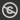Science, Maths & Technology
Free course

# Introduction to group theory## Course reviews

This free course is an introduction to group theory, one of the three main branches of pure mathematics. Section 1 looks at the set of symmetries of a two-dimensional figure which are then viewed as functions. Section 2 introduces an algebraic notation for recording symmetries and calculating composites and inverses of symmetries. Section 3 introduces definitions and looks at how to check axioms for a group. Section 4 looks at how to prove that some properties of groups already looked at are general properties shared by all groups. Section 5 extends the ideas of symmetry to three-dimensions.

## Course learning outcomes

After studying this course, you should be able to:

• explain what is meant by a symmetry of a plane figure
• find the composite of two symmetries
• find the inverse of a symmetry
• determine whether a given set and binary operation form a group by checking group axioms
• describe the symmetries of some bounded three-dimensional figures.

First Published: 08/12/2017

Updated: 18/04/2018

Skip Rate and Review